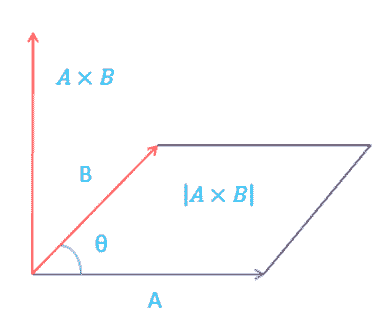### Calculate Product of Two Dimensional Vectors

Calculate Product of Two Dimensional Vectors (2D Vector)
The inner product or scalar product of two vectors can be defined as: A * B = |A| * |B| * Cos(theta) Where |A| and |B| represents the magnitudes of vectors A and B and (theta) is the angle between vectors A and B.Vector A: , Vector B: , Scalar Product:

Lets consider the two vector A and B for dot or scalar product.
The dot product is a form of multiplication that involves two vectors having the same number of components. To determine the dot product of two vectors, we always multiply like components, and find their sum.
Let consider value for vector A as (2 , 3) and B as (4 , 6)
The A*B = a1* b1 + a2* b2
= 2x4 + 3x6
= 8 + 18
= 26